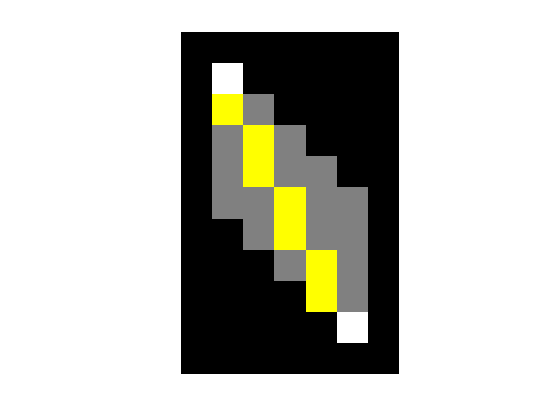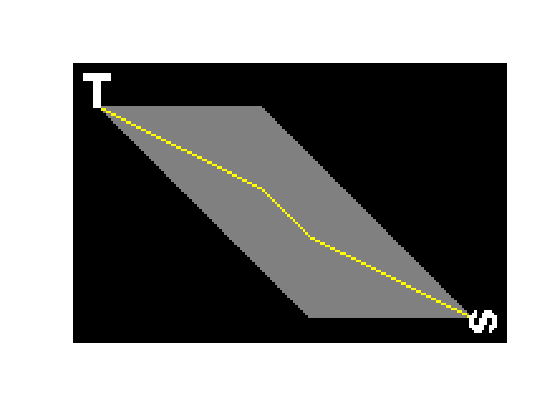# Exploring shortest paths – part 4

In my previous posts on Exploring shortest paths (November 1, November 26, and December 3), I have noted several times that there isn't a unique shortest path (in general) between one object and another in a binary image. To illustrate, here's a recap of the 'quasi-euclidean' example from last time.

bw = logical([ ...
0 0 0 0 0 0 0
0 1 0 0 0 0 0
0 0 0 0 0 0 0
0 0 0 0 0 0 0
0 0 0 0 0 0 0
0 0 0 0 0 0 0
0 0 0 0 0 0 0
0 0 0 0 0 0 0
0 0 0 0 0 0 0
0 0 0 0 0 1 0
0 0 0 0 0 0 0  ]);
L = bwlabel(bw);
bw1 = (L == 1);
bw2 = (L == 2);

D1 = bwdist(bw1, 'quasi-euclidean');
D2 = bwdist(bw2, 'quasi-euclidean');
D = D1 + D2;
D = round(D * 32) / 32;

paths = imregionalmin(D);
P = false(size(bw));
P = imoverlay(P, paths, [.5 .5 .5]);
P = imoverlay(P, bw, [1 1 1]);
imshow(P, 'InitialMagnification', 'fit')The gray pixels shown above all belong to one or more of the shortest paths between the two objects. So how do we pick one path?

The first idea I had was to thin the paths "blob" using the 'thin' option of bwmorph.

paths_thinned_once = bwmorph(paths, 'thin');
P = false(size(bw));
P = imoverlay(P, paths, [.5 .5 .5]);
P = imoverlay(P, paths_thinned_once, [1 1 0]);
P = imoverlay(P, bw, [1 1 1]);
imshow(P, 'InitialMagnification', 'fit')That seems promising. What if we keep thinning until convergence? You do that by passing inf as the repetition value to bwmorph.

paths_thinned_many = bwmorph(paths, 'thin', inf);
P = false(size(bw));
P = imoverlay(P, paths, [.5 .5 .5]);
P = imoverlay(P, paths_thinned_many, [1 1 0]);
P = imoverlay(P, bw, [1 1 1]);
imshow(P, 'InitialMagnification', 'fit')That looks great.

Let's try the whole sequence with a longer path.

url = 'https://blogs.mathworks.com/images/steve/2011/two-letters-cropped.png';
imshow(bw, 'InitialMagnification', 'fit')L = bwlabel(bw);
bw1 = (L == 1);
bw2 = (L == 2);

D1 = bwdist(bw1, 'quasi-euclidean');
D2 = bwdist(bw2, 'quasi-euclidean');
D = D1 + D2;
D = round(D * 32) / 32;

paths = imregionalmin(D);

paths_thinned_many = bwmorph(paths, 'thin', inf);
P = false(size(bw));
P = imoverlay(P, paths, [.5 .5 .5]);
P = imoverlay(P, paths_thinned_many, [1 1 0]);
P = imoverlay(P, bw, [1 1 1]);
imshow(P, 'InitialMagnification', 'fit')As before, the pixels belonging to any shortest path are shown in gray. The pixels in yellow belong to one particular path.

Next time I'll explain how to find shortest paths subject to constraints.

#### All the posts in this series

• the basic idea of finding shortest paths by adding two distance transforms together (part 1)
• the nonuniqueness of the shortest paths (part 2)
• handling floating-point round-off effects (part 3)
• using thinning to pick out a single path (part 4)
• using bwdistgeodesic to find shortest paths subject to constraint (part 5)

Published with MATLAB® 7.13

|